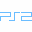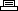Site SearchMLB SlugFest 2004Printer Friendly Version

```Cheat mode:
Press Square, Triangle, and Circle to change the icons
in the first, second, and third boxes respectively at
the match-up screen.

Effect                          Code
Bone bat                        Circle, Up
Maximum speed                   Circle(3), Left
Ice bat                         Circle(3), Up
Mace bat                        Circle(4), Left
Wiffle bat                      Circle(4), Right
Log bat                         Circle(4), Up
Spike bat                       Circle(5), Up
Maximum power                   Triangle(3), Left
Little League mode              Square, Circle(1), Down
Dolphin team                    Square, Circle(2), Down
Dwarf team                      Square, Circle(3), Down
Minotaur team                   Square, Triangle, Down
Tournament mode                 Square, Triangle, Circle(1), Down
Scorpion team                   Square, Triangle, Circle(2), Down
Gladiator team                  Square, Triangle, Circle(3), Down
Sub-Zero team                   Square, Triangle(2), Circle(2), Down
Extra time after plays          Square, Triangle(2), Circle(3), Up
Rodeo Clown team                Square, Triangle(3), Circle(2), Down
Bobble Head team                Square, Triangle(3), Circle(3), Down
Pinto team                      Square(2), Triangle, Right
Evil Clown team                 Square(2), Triangle, Circle(1), Down
Horse team                      Square(2), Triangle, Circle(1), Right
Eagle team                      Square(2), Triangle, Circle(2), Right
Lion team                       Square(2), Triangle(2), Right
Oshlan team                     Square(2), Triangle(2), Circle(2), Down
Todd McFarlane team             Square(2), Triangle(2), Circle(2), Right
Rivera team                     Square(2), Triangle(2), Circle(2), Up
Alien team                      Square(2), Triangle(3), Circle(1), Down
Napalitano team                 Square(2), Triangle(3), Circle(2), Down
Casey team                      Square(2), Triangle(3), Circle(3), Down
Softball                        Square(2), Triangle(4), Circle(2), Down
Rubber ball                     Square(2), Triangle(4), Circle(2), Up
Maximum batting                 Square(3), Left
Extended time for codes         Square(3), Circle(3), Up
Midway Park stadium             Square(3), Triangle(2), Circle Down
Atlantis stadium                Square(3), Triangle(2), Circle Left
Empire stadium                  Square(3), Triangle(2), Circle Right
Rocket Park                     Square(3), Triangle(2), Circle Up
Monument stadium                Square(3), Triangle(3), Circle(3), Down
Forbidden stadium               Square(3), Triangle(3), Circle(3), Left
Terry Fitzgerald team           Square(3), Triangle(3), Circle(3), Right
Coliseum stadium                Square(3), Triangle(3), Circle(3), Up
No fatigue                      Square(3), Triangle(4), Circle(3), Up
No contact mode                 Square(4), Triangle(3), Circle(3), Left
Unlimited turbo                 Square(4), Triangle(4), Circle(4), Down
```